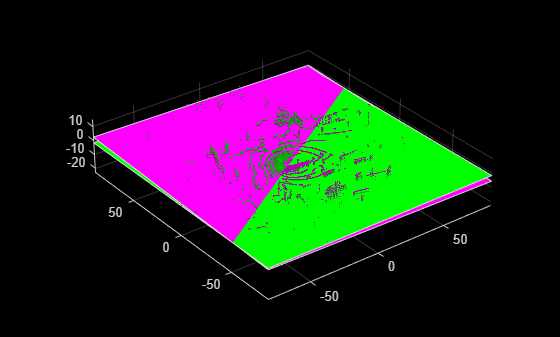# normalRotation

Compute transform for rotation of a normal to a plane

## Syntax

``normalRotation(model,referenceVector)``

## Description

example

````tform` = `normalRotation(model,referenceVector)` returns a `rigid3d` object `tform`, that specifies the transformation for a normal vector of a plane to the `referenceVector`. `model` is a `planeModel`. `referenceVector` is a 1-by-3 vector.```

## Examples

collapse all

`veloReader = velodyneFileReader('lidarData_ConstructionRoad.pcap','HDL32E');`

Read the first frame of lidar data.

```frameNumber = 1; ptCloud = readFrame(veloReader,frameNumber);```

Find the ground plane.

```maxDistance = 0.4; referenceVector = [0 0 1]; groundPlane = pcfitplane(ptCloud,maxDistance,referenceVector);```

Transform the ground plane such that it is parallel to the X-Y plane.

`tform = normalRotation(groundPlane,referenceVector);`

Transform the point cloud.

`ptCloudOut = pctransform(ptCloud,tform);`

Display the original and transformed point cloud.

```planeParams = groundPlane.Parameters * tform.T; transformedPlane = planeModel(planeParams); figure; pcshowpair(ptCloudOut,ptCloud); hold on; plot(groundPlane, 'Color', 'magenta'); plot(transformedPlane, 'Color', 'green');```## Input Arguments

collapse all

Parametric plane model returned by `planeModel`.

Reference vector, specified as a 1-by-3 vector.

### Objects

Introduced in R2020b